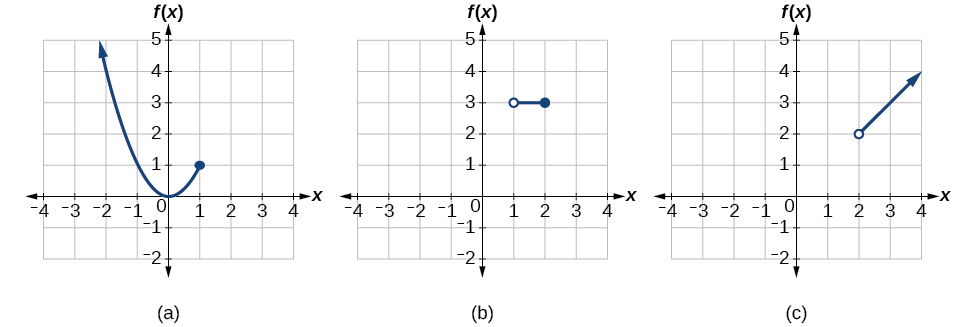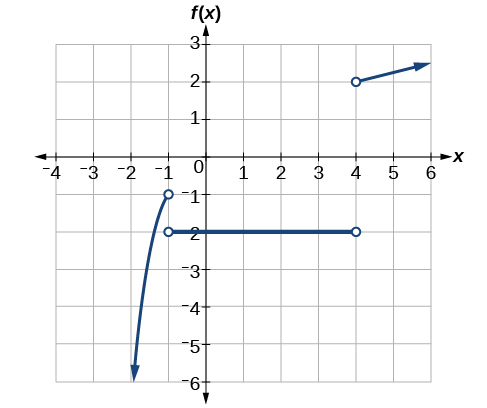# 3.2 Domain and range  (Page 7/11)

 Page 7 / 11

## Writing a piecewise function

A museum charges $5 per person for a guided tour with a group of 1 to 9 people or a fixed$50 fee for a group of 10 or more people. Write a function    relating the number of people, $\text{\hspace{0.17em}}n,\text{\hspace{0.17em}}$ to the cost, $\text{\hspace{0.17em}}C.$

Two different formulas will be needed. For n -values under 10, $\text{\hspace{0.17em}}C=5n.\text{\hspace{0.17em}}$ For values of $\text{\hspace{0.17em}}n\text{\hspace{0.17em}}$ that are 10 or greater, $\text{\hspace{0.17em}}C=50.$

$C\left(n\right)=\left\{\begin{array}{ccc}5n& \text{if}& 0

## Working with a piecewise function

A cell phone company uses the function below to determine the cost, $\text{\hspace{0.17em}}C,\text{\hspace{0.17em}}$ in dollars for $\text{\hspace{0.17em}}g\text{\hspace{0.17em}}$ gigabytes of data transfer.

$C\left(g\right)=\left\{\begin{array}{ccc}25& \text{if}& 0

Find the cost of using 1.5 gigabytes of data and the cost of using 4 gigabytes of data.

To find the cost of using 1.5 gigabytes of data, $\text{\hspace{0.17em}}C\left(1.5\right),\text{\hspace{0.17em}}$ we first look to see which part of the domain our input falls in. Because 1.5 is less than 2, we use the first formula.

$C\left(1.5\right)=\text{}25$

To find the cost of using 4 gigabytes of data, $\text{\hspace{0.17em}}C\left(4\right),\text{\hspace{0.17em}}$ we see that our input of 4 is greater than 2, so we use the second formula.

$C\left(4\right)=25+10\left(4-2\right)=\text{}45$

Given a piecewise function, sketch a graph.

1. Indicate on the x -axis the boundaries defined by the intervals on each piece of the domain.
2. For each piece of the domain, graph on that interval using the corresponding equation pertaining to that piece. Do not graph two functions over one interval because it would violate the criteria of a function.

## Graphing a piecewise function

Sketch a graph of the function.

$f\left(x\right)=\left\{\begin{array}{ccc}{x}^{2}& \text{if}& x\le 1\\ 3& \text{if}& 12\end{array}$

Each of the component functions is from our library of toolkit functions, so we know their shapes. We can imagine graphing each function and then limiting the graph to the indicated domain. At the endpoints of the domain, we draw open circles to indicate where the endpoint is not included because of a less-than or greater-than inequality; we draw a closed circle where the endpoint is included because of a less-than-or-equal-to or greater-than-or-equal-to inequality.

[link] shows the three components of the piecewise function graphed on separate coordinate systems.(a)   f ( x ) = x 2  if   x ≤ 1 ;   (b)   f ( x ) = 3  if 1<  x ≤ 2 ;   (c)   f ( x ) = x   if  x > 2

Now that we have sketched each piece individually, we combine them in the same coordinate plane. See [link] .

Graph the following piecewise function.

$f\left(x\right)=\left\{\begin{array}{ccc}{x}^{3}& \text{if}& x<-1\\ -2& \text{if}& -14\end{array}$Can more than one formula from a piecewise function be applied to a value in the domain?

No. Each value corresponds to one equation in a piecewise formula.

Access these online resources for additional instruction and practice with domain and range.

## Key concepts

• The domain of a function includes all real input values that would not cause us to attempt an undefined mathematical operation, such as dividing by zero or taking the square root of a negative number.
• The domain of a function can be determined by listing the input values of a set of ordered pairs. See [link] .
• The domain of a function can also be determined by identifying the input values of a function written as an equation. See [link] , [link] , and [link] .
• Interval values represented on a number line can be described using inequality notation, set-builder notation, and interval notation. See [link] .
• For many functions, the domain and range can be determined from a graph. See [link] and [link] .
• An understanding of toolkit functions can be used to find the domain and range of related functions. See [link] , [link] , and [link] .
• A piecewise function is described by more than one formula. See [link] and [link] .
• A piecewise function can be graphed using each algebraic formula on its assigned subdomain. See [link] .

sinx sin2x is linearly dependent
what is a reciprocal
The reciprocal of a number is 1 divided by a number. eg the reciprocal of 10 is 1/10 which is 0.1
Shemmy
Reciprocal is a pair of numbers that, when multiplied together, equal to 1. Example; the reciprocal of 3 is ⅓, because 3 multiplied by ⅓ is equal to 1
Jeza
each term in a sequence below is five times the previous term what is the eighth term in the sequence
I don't understand how radicals works pls
How look for the general solution of a trig function
stock therom F=(x2+y2) i-2xy J jaha x=a y=o y=b
sinx sin2x is linearly dependent
cr
root under 3-root under 2 by 5 y square
The sum of the first n terms of a certain series is 2^n-1, Show that , this series is Geometric and Find the formula of the n^th
cosA\1+sinA=secA-tanA
Wrong question
why two x + seven is equal to nineteen.
The numbers cannot be combined with the x
Othman
2x + 7 =19
humberto
2x +7=19. 2x=19 - 7 2x=12 x=6
Yvonne
because x is 6
SAIDI
what is the best practice that will address the issue on this topic? anyone who can help me. i'm working on my action research.
simplify each radical by removing as many factors as possible (a) √75
how is infinity bidder from undefined?
what is the value of x in 4x-2+3
give the complete question
Shanky
4x=3-2 4x=1 x=1+4 x=5 5x
Olaiya
hi can you give another equation I'd like to solve it
Daniel
what is the value of x in 4x-2+3
Olaiya
if 4x-2+3 = 0 then 4x = 2-3 4x = -1 x = -(1÷4) is the answer.
Jacob
4x-2+3 4x=-3+2 4×=-1 4×/4=-1/4
LUTHO
then x=-1/4
LUTHO
4x-2+3 4x=-3+2 4x=-1 4x÷4=-1÷4 x=-1÷4
LUTHO
A research student is working with a culture of bacteria that doubles in size every twenty minutes. The initial population count was  1350  bacteria. Rounding to five significant digits, write an exponential equation representing this situation. To the nearest whole number, what is the population size after  3  hours?
f(x)= 1350. 2^(t/20); where t is in hours.
MerkebByByBy Jonathan LongBy Megan EarhartBy John GabrieliBy David BourgeoisBy Rylee MinllicByBy Michael SagBy Richley CrapoBy OpenStaxBy OpenStax